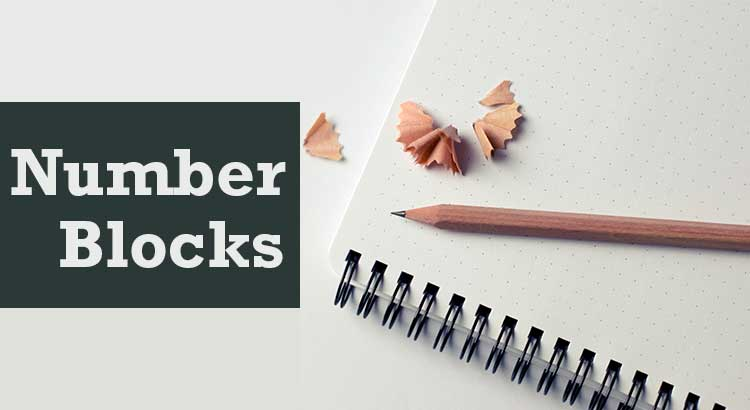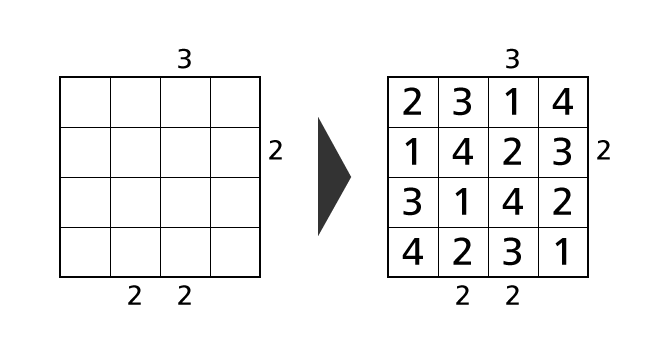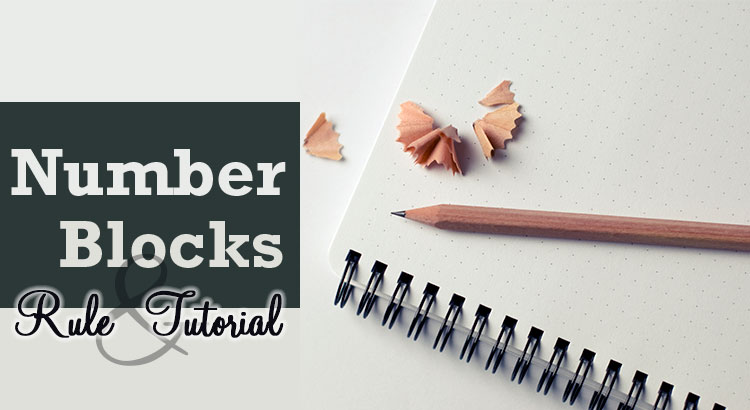## Introduction

Today’s puzzle is CalcBlocks. If you are new to CalcBlocks, please read Rule and Tutorial of CalcBlocks first. Previous puzzles are available from link buttons below (Puzzles will be refreshed). A PDF file for print is also available at the end of this article.

CalcBlocks shares the same rules with Sudoku that each column and each row contain each digit exactly once. In addition, a calculation result of each region by a specific operator is provided. Calculation ability is required compared to other kinds of puzzles.

Difficulty of puzzles depends on puzzle size or kinds of used operators. So it would be nice to try puzzles that contain only addition as an operator first.

We make all puzzles have only one solution.

## Today’s Puzzle

In this puzzle, results of addition of numbers in each region are provided as hints.## Introduction

Today’s puzzle is Sudoku 4×4. If you are new to Sudoku 4×4, please read Rule and Tutorial of Sudoku 4×4 first. Previous Sudoku puzzles are available from link buttons below (Puzzles will be refreshed). A PDF file for print is also available at the end of this article.

Sudoku 4×4 is a smaller size variant of Sudoku. Despite that size, it is more difficult than you may think and includes all the interesting parts of normal Sudoku. Sudoku 4×4 takes less time to solve. So it is suitable for killing time. I also recommend it to people whom normal Sudoku is too difficult for.

We make all puzzles have only one solution.

## Today’s Puzzle

### Problem 1## Today’s Puzzle

Today’s puzzle is Skyscrapers puzzle. If you are new to Skyscrapers puzzle, please read Rule and Tutorial of Skyscrapers Puzzle first. Previous puzzles are available from link buttons below (Puzzles will be refreshed). A PDF file for print is also available at the end of this article.

Skyscrapers puzzle shares the same rules with Sudoku that each column and each row contain each digit exactly once.

When we play Skyscrapers puzzle, we assume that each digit represents the height of each skyscraper. With that in mind, counts of skyscrapers or calculation results of heights of skyscrapers you can see from the specific direction are provided.

Understanding about permutation of numbers is required compared to other kinds of puzzles.

We make all puzzles have only one solution.

## Today’s Puzzle

### Count

In this puzzle, counts of skyscrapers you can see from each direction are provided as hints.## Introduction

Today’s puzzle is NumberBlocks. If you are new to NumberBlocks, please read Rule and Tutorial of NumberBlocks first. Previous puzzles are available from link buttons below (Puzzles will be refreshed). A PDF file for print is also available at the end of this article.

NumberBlocks is a variant of Sudoku puzzles. So we have to place digits under the constraint that each column, each row and each region (surrounded by thick lines) contain each digit exactly only once. NumberBlocks does not contain any calculations. People of all ages can play with them.

We make all puzzles have only one solution.

## Today’s Puzzle

### Problem 1## Introduction

When we play Skyscrapers Puzzle, we assume that we are watching the buildings with heights of 1–4 from above.Skyscrapers Puzzle is a puzzle game which we have to determine the place of numbers by using given hints under some rules.Here we explain the rule of Skyscrapers Puzzle with size 4×4 in detail for those who plays it for the first time. Then we demonstrate the solving process of an example puzzle.## Introduction

CalcBlocks is a puzzle game which we have to determine the place of numbers by using given hints under some rules. Here we explain the rule of CalcBlocks with size 4×4 in detail for those who plays it for the first time. Then we demonstrate the solving process of an example puzzle.

In explanations, we call a horizontal sequence of boxes row and a vertical sequence of boxes column. We describe a position of a box as (1,1) using a row and a column number. For example, we call the colored box in the following image box–(2,3).## Introduction

Sudoku 4×4 is a puzzle game which we have to determine the place of numbers by using given hints under some rules. Here we explain the rule of Sudoku 4×4 in detail for those who plays it for the first time. Then we demonstrate the solving process of an example puzzle.

In explanations, we call a horizontal sequence of boxes row and a vertical sequence of boxes column. We describe a position of a box as (1,1) using a row and a column number. For example, we call the colored box in the following image box–(2,3).